期刊菜单

Bilateral Filter Algorithm of Vein Image Based on FPGA
DOI: 10.12677/JISP.2019.83018, PDF, HTML, XML, 下载: 703  浏览: 2,382  科研立项经费支持

Abstract: The bilateral filter algorithm reduces noise in hand vein image while preserving edge details of vein. By implementing the bilateral filter algorithm on FPGA, features of FPGA such as parallel and pipeline processing can boost the calculation speed of the algorithm. While satisfying the real-time requirements of hand vein imaging system, this implementation can enhance the hand vein image for subsequent segmentation and extraction of vein.

1. 引言

2. 双边滤波算法

$\stackrel{¯}{\varphi }\left(\stackrel{¯}{{m}_{0}}\right)=\frac{1}{k\left({m}_{0}\right)}{\sum }_{m\in F}\varphi \left(m\right)\cdot s\left(\varphi \left({m}_{0}\right),\varphi \left(m\right)\right)\cdot c\left({m}_{0},m\right)$ (1)

$s\left(\varphi \left({m}_{0}\right),\varphi \left(m\right)\right)={e}^{-\frac{1}{2}{\left(\frac{‖\varphi \left({m}_{0}\right)-\varphi \left(m\right)‖}{{\sigma }_{ph}}\right)}^{2}}$ (2)

$c\left({m}_{0},m\right)={e}^{-\frac{1}{2}{\left(\frac{‖{m}_{0}-m‖}{{\sigma }_{c}}\right)}^{2}}$ (3)

$k\left({m}_{0}\right)={\sum }_{m\in F}S\left(\varphi \left({m}_{0}\right),\varphi \left(m\right)\right)\cdot c\left({m}_{0},m\right)$ (4)

3. 双边滤波算法的FPGA实现

3.1. 双边滤波处理步骤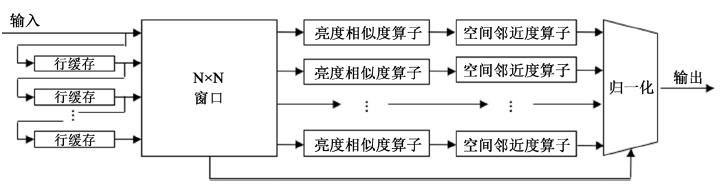Figure 1. Flow chart of algorithm process

3.2. 亮度相似度权重系数计算

1) 像素值的位宽N；

2) 参数σph

3) 权重系数的位宽W；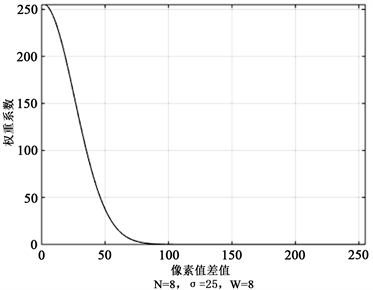Figure 2. Limitation of the number of weight coefficient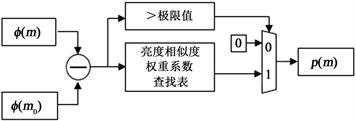Figure 3. Brightness similarity operato

3.3. 空间邻近度权重系数计算

3.4. 归一化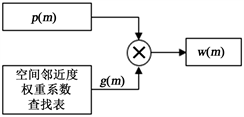Figure 4. Spatial proximity operator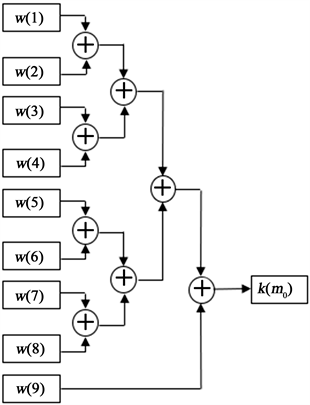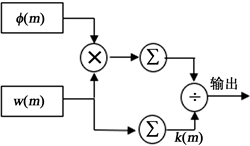Figure 6. Normalization of weight pixel value

4. 实验结果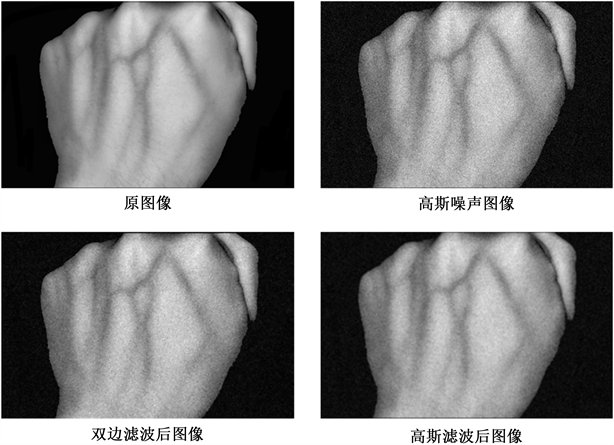Figure 7. Images filtered by bilateral filter and gauss filter

5. 结论

NOTES

*通讯作者。

  朱东莹, 吕勇, 沈俊杰. 基于近红外光的静脉深度估算研究[J]. 科技创新与应用, 2018(28): 18-21.  白书华. 基于FPGA的实时图像采集和去噪系统设计[J]. 航天器环境工程, 2016, 33(2): 163-166.  王玉灵. 基于双边滤波的图像处理算法研究[D]: [硕士学位论文]. 西安: 西安电子科技大学, 2010.  公羽, 吕志鹏. 基于双边滤波的点云数据预处理方法[J]. 测绘与空间地理信息, 2016, 39(1): 125-127.  乔峰, 张慧欣. 一种基于FPGA硬件求解函数的简化方法[J]. 电子科学技术, 2015, 2(5): 532-537.